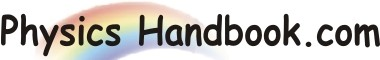HOME TOPICS DEFINITIONS TABLES LAWS INVENTIONS EXPERIMENTS QUIZ VIDEOS
 Faraday's First Law Of Electrolysis The mass of the substance librated or deposited on an electrode during electrolysis is directly proportional to the quantity of electric charge passed through the electrolyte. If m is the mass of a substance librated or deposited at an electrode due to the passage of charge q, then according to Faraday's law of electrolysis, m ∞ q    or    m  =  zq Where z is a constant of proportionality and is called electrochemical equivalent of the substance. It has the same charge which passes is due to a steady current I flowing for time t, then above equation can be written as: m  =  z x I x t      =  z x q if  q  =  1C, then m  =  z Hence electrochemical equivalent of a substance is the mass of the substance librated or deposited in electrolysis by the passage of 1 coulomb of charge.